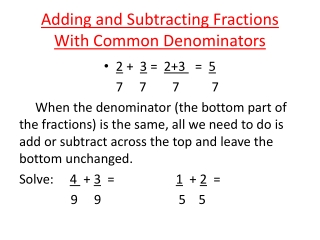DownloadDownload PresentationAdding and Subtracting Fractions With Common Denominators

# Adding and Subtracting Fractions With Common Denominators

Download Presentation## Adding and Subtracting Fractions With Common Denominators

- - - - - - - - - - - - - - - - - - - - - - - - - - - E N D - - - - - - - - - - - - - - - - - - - - - - - - - - -
##### Presentation Transcript

1. Adding and Subtracting Fractions With Common Denominators • 2 + 3 = 2+3 = 5 7 7 7 7 When the denominator (the bottom part of the fractions) is the same, all we need to do is add or subtract across the top and leave the bottom unchanged. Solve: 4 + 3 = 1 + 2 = 9 9 5 5

2. With Unlike Denominators • 1 + 2 = The first step is to find the lowest 3 5 common denominator. That is the smallest number that the two “bottoms” of the fractions will go into. In this case it is 15. We change the original question to two fractions with the same denominator (15) 1+ 21 = 52 = 6 so 5 + 6 = 11 3 5 3 15 5 15 15 15 15

3. Adding and Subtracting Fractions • Solve: • ¼ + 2/3 = • What is the lowest common denominator? • ¼ = and 2/3 = • So, rewrite the original question as:

4. Adding and Subtracting Fractions • Solve 1/6 + 2/5